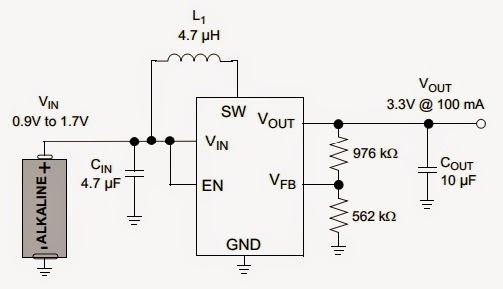## Monday, February 2, 2015

### Converter 1.2V/1.5V Single Cell to 3.3V Circuit Schematic using IC MCP1640

Eltronicschool. - Sometime we need 3.3 VDC to charge device. But we can not special cell that provide 3.3 volt. Maybe we can combine some cells with parallel and series sequence, but we think we can not get optimal 3.3 volt. So, to solve this problem we need electronic circuit schematic that can provide 3.3 volt.

In here we will show you Converter 1.2V/1.5V Single Cell to 3.3V Circuit Schematic using IC MCP1640. So, with only single cell 1.2V/1.5V we can get 3.3V easily. Special IC that used in this circuit schematic is MCP1640 that you can get datasheet of this IC here.

Circuit SchematicFigure 1. Converter 1.2V/1.5V Single Cell to 3.3V Circuit (Source:Electroschematic)

Component List
1. Capacitors
2. Inductors
3. IC MCP1640
Description

Circuit schematic like in figure 1 above is Converter 1.2V/1.5V Single Cell to 3.3V Circuit Schematic using IC MCP1640. This circuit can produce 3.3 volt output voltage. The output voltage can be adjusted to 3.3V (from 2.5V to 5V) by using this formula:

VOUT = 1.23V * [1 + (R1/R2)]

You need to connect the SHDN pin to Vin through a 1MΩ pull-up resistor. The output current of this circuit is more than 100mA. This circuit using single cell as input voltage 0.9 to 1.7 volt.